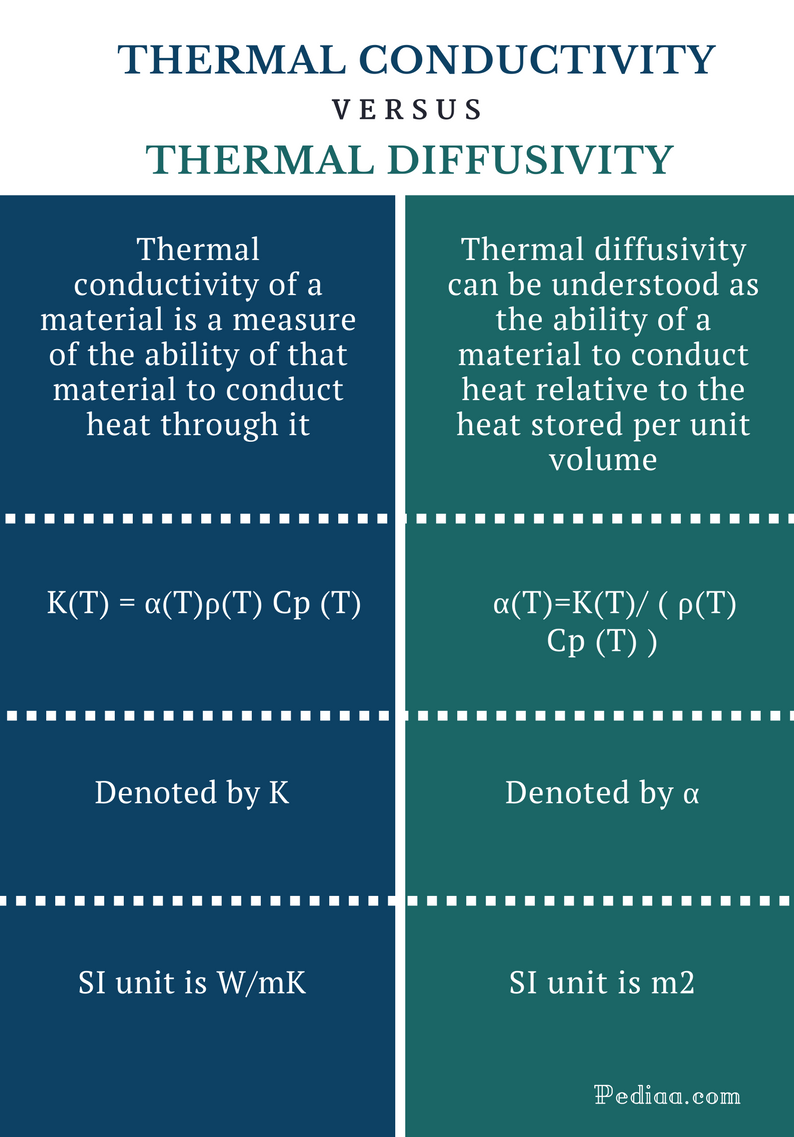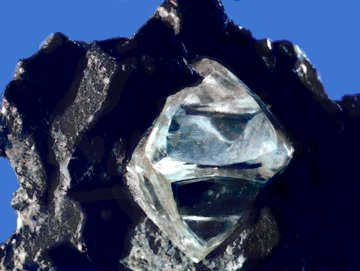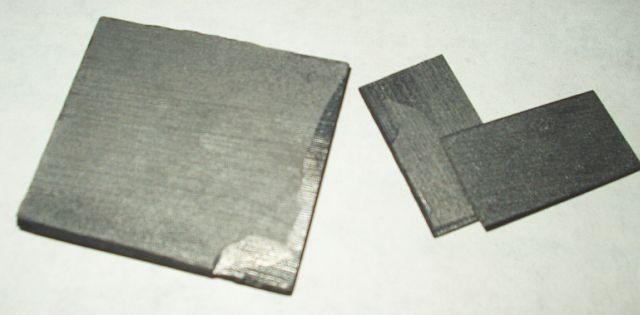# Difference Between Thermal conductivity and Thermal Diffusivity

## Main Difference – Thermal conductivity vs Thermal Diffusivity

Thermal conductivity and thermal diffusivity are two terms used in thermal and statistical physics. Thermal conductivity is a frequently used term in physics whereas thermal diffusivity is a rarely used term in thermal physics. The thermal conductivity of a material is a measure of the ability of that material to conduct heat through it. Thermal diffusivity of a material, on the other hand, is the thermal inertia of that material. This is the main difference between thermal conductivity and thermal diffusivity. Thermal conductivity is closely related to the thermal diffusivity. The relationship between the two quantities can be expressed as an equation.

1. What is Thermal conductivity? – Definition, Unit of Measurement, Formula, Properties of Thermal Conductors

2. What is Thermal Diffusivity? – Definition, Unit of Measurement, Formula, Properties

3. What is the difference between Thermal conductivity and Thermal Diffusivity?## What is Thermal Conductivity

In physics, thermal conductivity is the ability of a material to conduct heat. Thermal conductivity is denoted by the symbol K. The SI unit of measuring thermal conductivity is Watts per meter Kelvin (W/mK). The thermal conductivity of a given material often depends on the temperature and even the direction of heat transfer. According to the second law of thermodynamics, heat always flows from a hot region to a cold region. In other words, a net heat transfer needs a temperature gradient. Higher the thermal conductivity of a material, higher the rate of heat transfer across that material will be.

The reciprocal of the thermal conductivity of a given material is known as the thermal resistivity of that material. That means, higher the thermal conductivity, lower the thermal resistivity. Thermal conductivity (K) of a material can be expressed as;

K(T) = α (T)p (T) Cp(T)

Where, α(T)- Thermal diffusivity, p(T) – density, CpT- specific heat capacity

Materials such as diamond, Copper, Aluminium, and Silver have high thermal conductivities and are considered as good thermal conductors. Aluminum alloys are widely used as heat sinks especially in electronics. Materials such as wood, polyurethane, Alumina and polystyrene, on the other hand, have low thermal conductivity. Therefore, such materials are used as thermal insulators.

The thermal conductivity of a material can change when the phase of the material changes from solid to liquid, liquid to gas or vice versa. For instance, the thermal conductivity of ice changes when ice melts into water.

Good electrical conductors are usually good thermal conductors. However, Silver is a relatively weak thermal conductor even though it is a good electrical conductor.

Electrons are the main contributor to the thermal conductivity of metals whereas lattice vibrations or phonons are the main contributors to the thermal conductivity of nonmetals. In metals, the thermal conductivity is approximately proportional to the product of the electrical conductivity and the absolute temperature. However, the electrical conductivity of pure metals decreases when the temperature increases as the electrical resistance of pure metals increase with increasing temperature. As a result, the product of the electrical resistance and the absolute temperature as well as the thermal conductivity remain approximately constant with increasing or decreasing temperature.Diamond is one of the best thermal condutor around room temperature, having a thermal conductivity of more than 2,000 watts per meter per Kelvin.

## What is Thermal Diffusivity

Thermal diffusivity of a material is the thermal inertia of that material. It can be understood as the ability of a material to conduct heat, relative to the heat stored per unit volume.

The thermal diffusivity of a material can be defined as the thermal conductivity divided by the product of specific heat capacity and density. It can be expressed mathematically as;

α(T)= K(T)/(p(T)Cp(T))

α(T) = Thermal diffusivity

That means, higher the thermal diffusivity, higher the thermal conductivity. Therefore, materials having higher thermal diffusivity conduct heat quickly through them. The thermal diffusivity of a gas is highly sensitive to temperature as well as pressure. The SI unit of measuring thermal diffusivity is m2s-1.

Unlike thermal conductivity, thermal diffusivity is not a frequently used term. However, it is an important physical property of materials which helps to understand the ability of a material to conduct heat relative to the heat stored per unit volume.Pyrolytic graphite has a thermal diffusivity of 1.22 × 10−3 m2/s

## Difference Between Thermal conductivity and Thermal Diffusivity

### Definition:

Thermal Conductivity: Thermal conductivity of a material is a measure of the ability of that material to conduct heat through it.

Thermal Diffusivity: Thermal diffusivity can be understood as the ability of a material to conduct heat relative to the heat stored per unit volume.

### Formula for Calculation

Thermal conductivity (K) of a material can be expressed as;

K(T) = α(T)ρ(T) Cp (T)

Where, α(T) – Thermal diffusivity, ρ(T)- density, Cp (T)- specific heat capacity

Thermal diffusivity (α) of a material can be expressed in terms of thermal conductivity as;

α(T)=K(T)/( ρ(T) Cp (T) )

### Denoted by:

Thermal Conductivity: K

Thermal Diffusivity: α

### SI unit:

Thermal Conductivity: W/mK

Thermal Diffusivity: m2.

### Dimensions

Thermal Conductivity: M1L1T−3Θ−1

Thermal Diffusivity: L2.

Image Courtesy:

“Rough Diamond” By Unknown USGS employee – Original source: USGS “Minerals in Your World” website. Direct image link:  (Public Domain) via Commons Wikimedia

“Pyrolytic graphite” (CC BY-SA 3.0) via Commons Wikimedia